# Bernoulli and binomial distribution¶

The Bernoulli distribution of mean $p\in[0,1]$ is defined as the distribution on $\{0,1\}$ such that $\mathbb{P}(X=1) = p$ and $\mathbb{P}(X=0) = 1-p$.

If $X$ follows a Binomial distribution of mean $p\in[0,1]$ and $n$ samples, $X$ is defined as the sum of $n$ independent and identically distributed (iid) samples from a Bernoulli distribution of mean $p$, that is $X\in\{0,\dots,n\}$ ($X\in\mathbb{N}$) and $\forall k\in\{0,\dots,n\}, \mathbb{P}(X=k) = {n \choose k} p^k (1-p)^{n-k}$.

## Requirements¶

Let's import the modules required for this notebook.

In :
import numpy as np
import matplotlib.pyplot as plt

In :
%load_ext cython

In :
%load_ext watermark
%watermark -a "Lilian Besson (Naereen)" -i -v -p numpy,matplotlib,cython

Lilian Besson (Naereen) 2019-02-28T14:09:13+01:00

CPython 3.6.7
IPython 7.2.0

numpy 1.15.4
matplotlib 3.0.2
cython 0.29.2


## A naive generator¶

Using the pseudo-random generator of (float) random numbers in $[0,1]$ from the random or numpy.random module, we can easily generate a sample from a Bernoulli distribution.

In :
import random

def uniform_01() -> float:
return random.random()

In :
[ uniform_01() for _ in range(5) ]

Out:
[0.02240955612218365,
0.7770015834088284,
0.8358045673716832,
0.4095374878779069,
0.987872833694527]

It's very quick now:

In :
def bernoulli(p: float) -> int:
return 1 if uniform_01() <= p else 0

In :
[ bernoulli(0) for _ in range(5) ]

Out:
[0, 0, 0, 0, 0]
In :
[ bernoulli(0.12345) for _ in range(5) ]

Out:
[1, 0, 0, 0, 0]
In :
[ bernoulli(1) for _ in range(5) ]

Out:
[1, 1, 1, 1, 1]

So we can naively generate samples from a Binomial distribution by summing iid samples generated using this bernoulli function.

In :
def naive_binomial(n: int, p: float) -> int:
result = 0
for k in range(n):  # sum of n iid samples from Bernoulli(p)
result += bernoulli(p)
return result


For example :

In :
[ naive_binomial(10, 0.1) for _ in range(5) ]

Out:
[0, 1, 2, 0, 1]
In :
[ naive_binomial(10, 0.5) for _ in range(5) ]

Out:
[8, 4, 6, 5, 8]
In :
[ naive_binomial(10, 0.9) for _ in range(5) ]

Out:
[10, 9, 8, 10, 10]

We can quickly illustrate the generated distribution, to check it has the correct "shape":

In :
m = 1000
n = 10
p = 0.12345
X = [ naive_binomial(n, p) for _ in range(m) ]
plt.figure()
plt.hist(X)
plt.title(f"{m} samples from a Binomial distribution with n = {n} and p = {p}.")
plt.show()

Out:
<Figure size 432x288 with 0 Axes>
Out:
(array([254.,   0., 378.,   0., 248.,   0.,  92.,   0.,  24.,   4.]),
array([0. , 0.5, 1. , 1.5, 2. , 2.5, 3. , 3.5, 4. , 4.5, 5. ]),
<a list of 10 Patch objects>)
Out:
Text(0.5, 1.0, '1000 samples from a Binomial distribution with n = 10 and p = 0.12345.')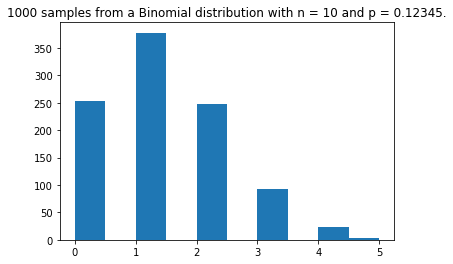In :
m = 1000
n = 10
p = 0.5
X = [ naive_binomial(n, p) for _ in range(m) ]
plt.figure()
plt.hist(X)
plt.title(f"{m} samples from a Binomial distribution with n = {n} and p = {p}.")
plt.show()

Out:
<Figure size 432x288 with 0 Axes>
Out:
(array([  1.,  10.,  41.,  98., 215., 236., 219., 116.,  51.,  13.]),
array([ 0.,  1.,  2.,  3.,  4.,  5.,  6.,  7.,  8.,  9., 10.]),
<a list of 10 Patch objects>)
Out:
Text(0.5, 1.0, '1000 samples from a Binomial distribution with n = 10 and p = 0.5.')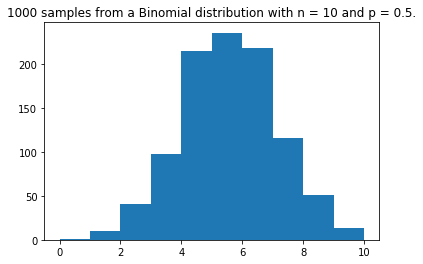In :
m = 1000
n = 10
p = 0.98765
X = [ naive_binomial(n, p) for _ in range(m) ]
plt.figure()
plt.hist(X)
plt.title(f"{m} samples from a Binomial distribution with n = {n} and p = {p}.")
plt.show()

Out:
<Figure size 432x288 with 0 Axes>
Out:
(array([  1.,   0.,   0.,   5.,   0.,   0., 101.,   0.,   0., 893.]),
array([ 7. ,  7.3,  7.6,  7.9,  8.2,  8.5,  8.8,  9.1,  9.4,  9.7, 10. ]),
<a list of 10 Patch objects>)
Out:
Text(0.5, 1.0, '1000 samples from a Binomial distribution with n = 10 and p = 0.98765.')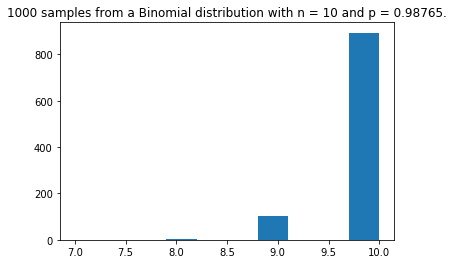## The generator included in numpy.random¶

In :
def numpy_binomial(n: int, p: float) -> int:
return np.random.binomial(n, p)


Let's try this out:

In :
[ numpy_binomial(10, 0.1) for _ in range(5) ]

Out:
[1, 1, 0, 1, 1]
In :
[ numpy_binomial(10, 0.5) for _ in range(5) ]

Out:
[4, 6, 7, 6, 5]
In :
[ numpy_binomial(10, 0.9) for _ in range(5) ]

Out:
[9, 9, 10, 8, 9]

Let's plot this out also.

In :
m = 1000
n = 10
p = 0.12345
X = [ numpy_binomial(n, p) for _ in range(m) ]
plt.figure()
plt.hist(X)
plt.title(f"{m} samples from a Binomial distribution with n = {n} and p = {p}.")
plt.show()

Out:
<Figure size 432x288 with 0 Axes>
Out:
(array([288., 343.,   0., 245.,   0.,  96.,  22.,   0.,   5.,   1.]),
array([0. , 0.6, 1.2, 1.8, 2.4, 3. , 3.6, 4.2, 4.8, 5.4, 6. ]),
<a list of 10 Patch objects>)
Out:
Text(0.5, 1.0, '1000 samples from a Binomial distribution with n = 10 and p = 0.12345.')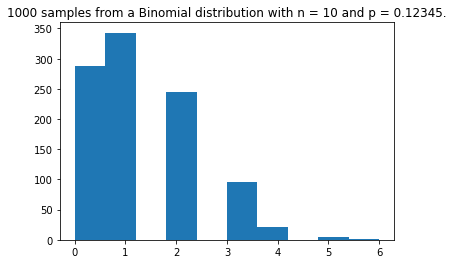In :
m = 1000
n = 10
p = 0.5
X = [ naive_binomial(n, p) for _ in range(m) ]
plt.figure()
plt.hist(X)
plt.title(f"{m} samples from a Binomial distribution with n = {n} and p = {p}.")
plt.show()

Out:
<Figure size 432x288 with 0 Axes>
Out:
(array([  2.,  10.,  46., 106., 218., 230., 211., 114.,  51.,  12.]),
array([ 0.,  1.,  2.,  3.,  4.,  5.,  6.,  7.,  8.,  9., 10.]),
<a list of 10 Patch objects>)
Out:
Text(0.5, 1.0, '1000 samples from a Binomial distribution with n = 10 and p = 0.5.')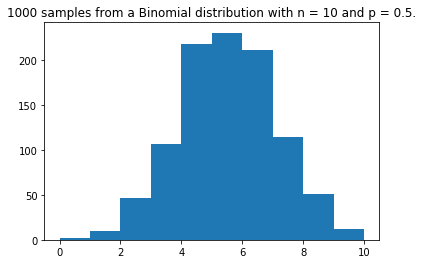In :
m = 1000
n = 10
p = 0.98765
X = [ naive_binomial(n, p) for _ in range(m) ]
plt.figure()
plt.hist(X)
plt.title(f"{m} samples from a Binomial distribution with n = {n} and p = {p}.")
plt.show()

Out:
<Figure size 432x288 with 0 Axes>
Out:
(array([  7.,   0.,   0.,   0.,   0., 105.,   0.,   0.,   0., 888.]),
array([ 8. ,  8.2,  8.4,  8.6,  8.8,  9. ,  9.2,  9.4,  9.6,  9.8, 10. ]),
<a list of 10 Patch objects>)
Out:
Text(0.5, 1.0, '1000 samples from a Binomial distribution with n = 10 and p = 0.98765.')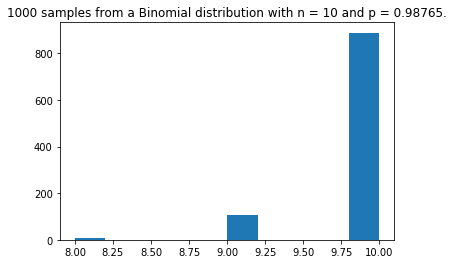## An efficient generator using the inverse transform method¶

1. We start by computing the binomial coefficients and then the probability $\mathbb{P}(X=k)$ for $k\in\{0,\dots,n\}$, if $X\sim Bin(n, p)$, and,
2. Then use this to write a generator of Binomial-distributed random values.
3. This function is then simplified to inline all computations.
4. We propose a fast and simple Cython implementation, to be as efficient as possible, and hopefully comparably efficient when compared against the implementation in Numpy.

### Explicit computation of the probabilities¶

In :
def binomial_coefficient(n: int, k: int) -> int:
"""From https://en.wikipedia.org/wiki/Binomial_coefficient#Binomial_coefficient_in_programming_languages"""
if k < 0 or k > n:
return 0
if k == 0 or k == n:
return 1
k = min(k, n - k) # take advantage of symmetry
c = 1
for i in range(k):
c = (c * (n - i)) / (i + 1)
return c

In :
def proba_binomial(n: int, p: float, k: int) -> float:
"""Compute {n \choose k} p^k (1-p)^(n-k)"""
q = 1.0 - p
return binomial_coefficient(n, k) * p**k * q**(n-k)


### First function using the inversion method¶

This first function is a generic implementation of the discrete inverse transform method. For more details, see the Wikipedia page.

Inverse transformation sampling takes uniform samples of a number $u$ between $0$ and $1$, interpreted as a probability, and then returns the largest number $x$ from the domain of the distribution $\mathbb{P}(X)$ such that $\mathbb{P}(-\infty <X<x)\leq u$.

In :
# a generic function
from typing import Callable

def inversion_method(compute_proba: Callable[[int], int], xmax: int, xmin: int =0) -> int:
probas = [ compute_proba(x) for x in range(xmin, xmax + 1) ]
result = xmin
current_proba = 0
one_uniform_sample = uniform_01()
while current_proba <= one_uniform_sample:
current_proba += probas[result]
result += 1
return result - 1

In :
def first_inversion_binomial(n: int, p: float) -> int:
def compute_proba(x):
return proba_binomial(n, p, x)
xmax = n
xmin = 0
return inversion_method(compute_proba, xmax, xmin=xmin)


Let's try out.

In :
[ first_inversion_binomial(10, 0.1) for _ in range(5) ]

Out:
[1, 0, 0, 1, 1]
In :
[ first_inversion_binomial(10, 0.5) for _ in range(5) ]

Out:
[5, 3, 4, 4, 5]
In :
[ first_inversion_binomial(10, 0.9) for _ in range(5) ]

Out:
[10, 9, 9, 8, 8]

It seems to work as wanted!

### Simplified code of the inversion method¶

The previous function as a few weaknesses: it stores the $n+1$ values of $\mathbb{P}(X=k)$ before hand, it computes all of them even if the for loop of the inversion method stops in average before the end (in average, it takes $np$ steps, which can be much smaller than $n$ for small $p$). Furthermore, the computations of both the binomial coefficients and the values $p^k (1-p)^{n-k}$ is using powers and not iterative multiplications, leading to more rounding errors.

We can solve all these issues by inlining all the computations.

In :
def inversion_binomial(n: int, p: float) -> int:
if p <= 1e-10:
return 0
if p >= 1 - 1e-10:
return n
if p > 0.5:  # speed up by computing for q and then substracting
return n - inversion_binomial(n, 1.0 - p)
result = 0
q = 1.0 - p
current_proba = q**n
cum_proba = current_proba
one_uniform_sample = uniform_01()
while cum_proba <= one_uniform_sample:
current_proba *= (p * (n - result)) / (q * (result + 1))
cum_proba += current_proba
result += 1
return result


Let's try out.

In :
[ inversion_binomial(10, 0.1) for _ in range(5) ]

Out:
[1, 2, 1, 2, 3]
In :
[ inversion_binomial(10, 0.5) for _ in range(5) ]

Out:
[4, 6, 5, 4, 4]
In :
[ inversion_binomial(10, 0.9) for _ in range(5) ]

Out:
[10, 10, 7, 10, 10]

It seems to work as wanted!

And now the storage is indeed $O(1)$, and the computation time is $O(x)$ if the return value is $x$, so the mean computation time is $O(np)$.

Note that if $p=1/2$, then $O(np) = O(n/2) = O(n)$, and thus this improved method using the inversion method is (asymptotically) as costly as the naive method (the first method which consists of summing $n$ iid samples from a Bernoulli of mean $p$).

Let's plot this out also.

In :
m = 1000
n = 10
p = 0.12345
X = [ inversion_binomial(n, p) for _ in range(m) ]
plt.figure()
plt.hist(X)
plt.title(f"{m} samples from a Binomial distribution with n = {n} and p = {p}.")
plt.show()

Out:
<Figure size 432x288 with 0 Axes>
Out:
(array([261., 367.,   0., 248.,   0.,  96.,  27.,   0.,   0.,   1.]),
array([0. , 0.6, 1.2, 1.8, 2.4, 3. , 3.6, 4.2, 4.8, 5.4, 6. ]),
<a list of 10 Patch objects>)
Out:
Text(0.5, 1.0, '1000 samples from a Binomial distribution with n = 10 and p = 0.12345.')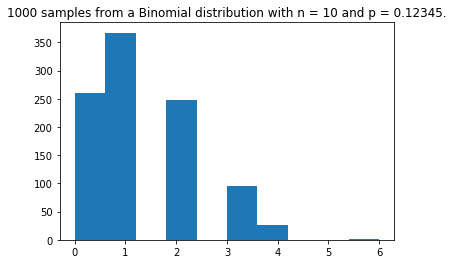In :
m = 1000
n = 10
p = 0.5
X = [ inversion_binomial(n, p) for _ in range(m) ]
plt.figure()
plt.hist(X)
plt.title(f"{m} samples from a Binomial distribution with n = {n} and p = {p}.")
plt.show()

Out:
<Figure size 432x288 with 0 Axes>
Out:
(array([  1.,  10.,  52., 115., 208., 239., 205., 110.,  49.,  11.]),
array([0. , 0.9, 1.8, 2.7, 3.6, 4.5, 5.4, 6.3, 7.2, 8.1, 9. ]),
<a list of 10 Patch objects>)
Out:
Text(0.5, 1.0, '1000 samples from a Binomial distribution with n = 10 and p = 0.5.')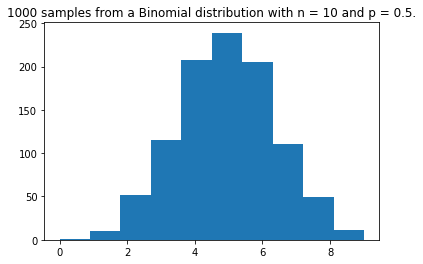In :
m = 1000
n = 10
p = 0.98765
X = [ inversion_binomial(n, p) for _ in range(m) ]
plt.figure()
plt.hist(X)
plt.title(f"{m} samples from a Binomial distribution with n = {n} and p = {p}.")
plt.show()

Out:
<Figure size 432x288 with 0 Axes>
Out:
(array([  7.,   0.,   0.,   0.,   0., 113.,   0.,   0.,   0., 880.]),
array([ 8. ,  8.2,  8.4,  8.6,  8.8,  9. ,  9.2,  9.4,  9.6,  9.8, 10. ]),
<a list of 10 Patch objects>)
Out:
Text(0.5, 1.0, '1000 samples from a Binomial distribution with n = 10 and p = 0.98765.')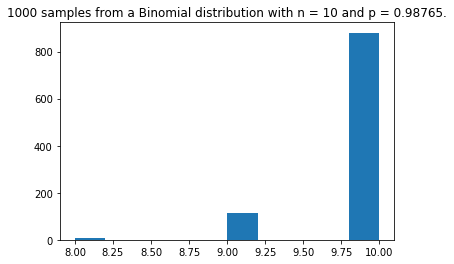### In Cython¶

In :
%load_ext cython

The cython extension is already loaded. To reload it, use:

In :
%%cython --annotate

import random

def cython_inversion_binomial(int n, double p) -> int:
if p <= 1e-9:
return 0
if p >= 1 - 1e-9:
return n
if p > 0.5:  # speed up by computing for q and then substracting
return n - cython_inversion_binomial(n, 1.0 - p)
cdef int result = 0
cdef double q = 1.0 - p
cdef double current_proba = q**n
cdef double cum_proba = current_proba
cdef double one_uniform_sample = random.random()
while cum_proba < one_uniform_sample:
current_proba *= (p * (n - result)) / (q * (result + 1))
cum_proba += current_proba
result += 1
return result

Out:

Generated by Cython 0.29.2

Yellow lines hint at Python interaction.
Click on a line that starts with a "+" to see the C code that Cython generated for it.

 01:
+02: import random
  __pyx_t_1 = __Pyx_Import(__pyx_n_s_random, 0, 0); if (unlikely(!__pyx_t_1)) __PYX_ERR(0, 2, __pyx_L1_error)
__Pyx_GOTREF(__pyx_t_1);
if (PyDict_SetItem(__pyx_d, __pyx_n_s_random, __pyx_t_1) < 0) __PYX_ERR(0, 2, __pyx_L1_error)
__Pyx_DECREF(__pyx_t_1); __pyx_t_1 = 0;

 03:
+04: def cython_inversion_binomial(int n, double p) -> int:
/* Python wrapper */
static PyObject *__pyx_pw_46_cython_magic_beb04c003b39b7afc4e8a1cfd3d3afad_1cython_inversion_binomial(PyObject *__pyx_self, PyObject *__pyx_args, PyObject *__pyx_kwds); /*proto*/
static PyObject *__pyx_pw_46_cython_magic_beb04c003b39b7afc4e8a1cfd3d3afad_1cython_inversion_binomial(PyObject *__pyx_self, PyObject *__pyx_args, PyObject *__pyx_kwds) {
int __pyx_v_n;
double __pyx_v_p;
PyObject *__pyx_r = 0;
__Pyx_RefNannyDeclarations
__Pyx_RefNannySetupContext("cython_inversion_binomial (wrapper)", 0);
{
static PyObject **__pyx_pyargnames[] = {&__pyx_n_s_n,&__pyx_n_s_p,0};
PyObject* values = {0,0};
if (unlikely(__pyx_kwds)) {
Py_ssize_t kw_args;
const Py_ssize_t pos_args = PyTuple_GET_SIZE(__pyx_args);
switch (pos_args) {
case  2: values = PyTuple_GET_ITEM(__pyx_args, 1);
CYTHON_FALLTHROUGH;
case  1: values = PyTuple_GET_ITEM(__pyx_args, 0);
CYTHON_FALLTHROUGH;
case  0: break;
default: goto __pyx_L5_argtuple_error;
}
kw_args = PyDict_Size(__pyx_kwds);
switch (pos_args) {
case  0:
if (likely((values = __Pyx_PyDict_GetItemStr(__pyx_kwds, __pyx_n_s_n)) != 0)) kw_args--;
else goto __pyx_L5_argtuple_error;
CYTHON_FALLTHROUGH;
case  1:
if (likely((values = __Pyx_PyDict_GetItemStr(__pyx_kwds, __pyx_n_s_p)) != 0)) kw_args--;
else {
__Pyx_RaiseArgtupleInvalid("cython_inversion_binomial", 1, 2, 2, 1); __PYX_ERR(0, 4, __pyx_L3_error)
}
}
if (unlikely(kw_args > 0)) {
if (unlikely(__Pyx_ParseOptionalKeywords(__pyx_kwds, __pyx_pyargnames, 0, values, pos_args, "cython_inversion_binomial") < 0)) __PYX_ERR(0, 4, __pyx_L3_error)
}
} else if (PyTuple_GET_SIZE(__pyx_args) != 2) {
goto __pyx_L5_argtuple_error;
} else {
values = PyTuple_GET_ITEM(__pyx_args, 0);
values = PyTuple_GET_ITEM(__pyx_args, 1);
}
__pyx_v_n = __Pyx_PyInt_As_int(values); if (unlikely((__pyx_v_n == (int)-1) && PyErr_Occurred())) __PYX_ERR(0, 4, __pyx_L3_error)
__pyx_v_p = __pyx_PyFloat_AsDouble(values); if (unlikely((__pyx_v_p == (double)-1) && PyErr_Occurred())) __PYX_ERR(0, 4, __pyx_L3_error)
}
goto __pyx_L4_argument_unpacking_done;
__pyx_L5_argtuple_error:;
__Pyx_RaiseArgtupleInvalid("cython_inversion_binomial", 1, 2, 2, PyTuple_GET_SIZE(__pyx_args)); __PYX_ERR(0, 4, __pyx_L3_error)
__pyx_L3_error:;
__Pyx_RefNannyFinishContext();
return NULL;
__pyx_L4_argument_unpacking_done:;

/* function exit code */
__Pyx_RefNannyFinishContext();
return __pyx_r;
}

static PyObject *__pyx_pf_46_cython_magic_beb04c003b39b7afc4e8a1cfd3d3afad_cython_inversion_binomial(CYTHON_UNUSED PyObject *__pyx_self, int __pyx_v_n, double __pyx_v_p) {
int __pyx_v_result;
double __pyx_v_q;
double __pyx_v_current_proba;
double __pyx_v_cum_proba;
double __pyx_v_one_uniform_sample;
PyObject *__pyx_r = NULL;
__Pyx_RefNannyDeclarations
__Pyx_RefNannySetupContext("cython_inversion_binomial", 0);
/* … */
/* function exit code */
__pyx_L1_error:;
__Pyx_XDECREF(__pyx_t_2);
__Pyx_XDECREF(__pyx_t_3);
__Pyx_XDECREF(__pyx_t_4);
__Pyx_XDECREF(__pyx_t_5);
__Pyx_XDECREF(__pyx_t_6);
__Pyx_XDECREF(__pyx_t_7);
__Pyx_XDECREF(__pyx_t_9);
__pyx_r = NULL;
__pyx_L0:;
__Pyx_XGIVEREF(__pyx_r);
__Pyx_RefNannyFinishContext();
return __pyx_r;
}
/* … */
__pyx_tuple_ = PyTuple_Pack(7, __pyx_n_s_n, __pyx_n_s_p, __pyx_n_s_result, __pyx_n_s_q, __pyx_n_s_current_proba, __pyx_n_s_cum_proba, __pyx_n_s_one_uniform_sample); if (unlikely(!__pyx_tuple_)) __PYX_ERR(0, 4, __pyx_L1_error)
__Pyx_GOTREF(__pyx_tuple_);
__Pyx_GIVEREF(__pyx_tuple_);
/* … */
__pyx_t_1 = PyCFunction_NewEx(&__pyx_mdef_46_cython_magic_beb04c003b39b7afc4e8a1cfd3d3afad_1cython_inversion_binomial, NULL, __pyx_n_s_cython_magic_beb04c003b39b7afc4); if (unlikely(!__pyx_t_1)) __PYX_ERR(0, 4, __pyx_L1_error)
__Pyx_GOTREF(__pyx_t_1);
if (PyDict_SetItem(__pyx_d, __pyx_n_s_cython_inversion_binomial, __pyx_t_1) < 0) __PYX_ERR(0, 4, __pyx_L1_error)
__Pyx_DECREF(__pyx_t_1); __pyx_t_1 = 0;

+05:     if p <= 1e-9:
  __pyx_t_1 = ((__pyx_v_p <= 1e-9) != 0);
if (__pyx_t_1) {
/* … */
}

+06:         return 0
    __Pyx_XDECREF(__pyx_r);
__Pyx_INCREF(__pyx_int_0);
__pyx_r = __pyx_int_0;
goto __pyx_L0;

+07:     if p >= 1 - 1e-9:
  __pyx_t_1 = ((__pyx_v_p >= (1.0 - 1e-9)) != 0);
if (__pyx_t_1) {
/* … */
}

+08:         return n
    __Pyx_XDECREF(__pyx_r);
__pyx_t_2 = __Pyx_PyInt_From_int(__pyx_v_n); if (unlikely(!__pyx_t_2)) __PYX_ERR(0, 8, __pyx_L1_error)
__Pyx_GOTREF(__pyx_t_2);
__pyx_r = __pyx_t_2;
__pyx_t_2 = 0;
goto __pyx_L0;

+09:     if p > 0.5:  # speed up by computing for q and then substracting
  __pyx_t_1 = ((__pyx_v_p > 0.5) != 0);
if (__pyx_t_1) {
/* … */
}

+10:         return n - cython_inversion_binomial(n, 1.0 - p)
    __Pyx_XDECREF(__pyx_r);
__pyx_t_2 = __Pyx_PyInt_From_int(__pyx_v_n); if (unlikely(!__pyx_t_2)) __PYX_ERR(0, 10, __pyx_L1_error)
__Pyx_GOTREF(__pyx_t_2);
__Pyx_GetModuleGlobalName(__pyx_t_4, __pyx_n_s_cython_inversion_binomial); if (unlikely(!__pyx_t_4)) __PYX_ERR(0, 10, __pyx_L1_error)
__Pyx_GOTREF(__pyx_t_4);
__pyx_t_5 = __Pyx_PyInt_From_int(__pyx_v_n); if (unlikely(!__pyx_t_5)) __PYX_ERR(0, 10, __pyx_L1_error)
__Pyx_GOTREF(__pyx_t_5);
__pyx_t_6 = PyFloat_FromDouble((1.0 - __pyx_v_p)); if (unlikely(!__pyx_t_6)) __PYX_ERR(0, 10, __pyx_L1_error)
__Pyx_GOTREF(__pyx_t_6);
__pyx_t_7 = NULL;
__pyx_t_8 = 0;
if (CYTHON_UNPACK_METHODS && unlikely(PyMethod_Check(__pyx_t_4))) {
__pyx_t_7 = PyMethod_GET_SELF(__pyx_t_4);
if (likely(__pyx_t_7)) {
PyObject* function = PyMethod_GET_FUNCTION(__pyx_t_4);
__Pyx_INCREF(__pyx_t_7);
__Pyx_INCREF(function);
__Pyx_DECREF_SET(__pyx_t_4, function);
__pyx_t_8 = 1;
}
}
#if CYTHON_FAST_PYCALL
if (PyFunction_Check(__pyx_t_4)) {
PyObject *__pyx_temp = {__pyx_t_7, __pyx_t_5, __pyx_t_6};
__pyx_t_3 = __Pyx_PyFunction_FastCall(__pyx_t_4, __pyx_temp+1-__pyx_t_8, 2+__pyx_t_8); if (unlikely(!__pyx_t_3)) __PYX_ERR(0, 10, __pyx_L1_error)
__Pyx_XDECREF(__pyx_t_7); __pyx_t_7 = 0;
__Pyx_GOTREF(__pyx_t_3);
__Pyx_DECREF(__pyx_t_5); __pyx_t_5 = 0;
__Pyx_DECREF(__pyx_t_6); __pyx_t_6 = 0;
} else
#endif
#if CYTHON_FAST_PYCCALL
if (__Pyx_PyFastCFunction_Check(__pyx_t_4)) {
PyObject *__pyx_temp = {__pyx_t_7, __pyx_t_5, __pyx_t_6};
__pyx_t_3 = __Pyx_PyCFunction_FastCall(__pyx_t_4, __pyx_temp+1-__pyx_t_8, 2+__pyx_t_8); if (unlikely(!__pyx_t_3)) __PYX_ERR(0, 10, __pyx_L1_error)
__Pyx_XDECREF(__pyx_t_7); __pyx_t_7 = 0;
__Pyx_GOTREF(__pyx_t_3);
__Pyx_DECREF(__pyx_t_5); __pyx_t_5 = 0;
__Pyx_DECREF(__pyx_t_6); __pyx_t_6 = 0;
} else
#endif
{
__pyx_t_9 = PyTuple_New(2+__pyx_t_8); if (unlikely(!__pyx_t_9)) __PYX_ERR(0, 10, __pyx_L1_error)
__Pyx_GOTREF(__pyx_t_9);
if (__pyx_t_7) {
__Pyx_GIVEREF(__pyx_t_7); PyTuple_SET_ITEM(__pyx_t_9, 0, __pyx_t_7); __pyx_t_7 = NULL;
}
__Pyx_GIVEREF(__pyx_t_5);
PyTuple_SET_ITEM(__pyx_t_9, 0+__pyx_t_8, __pyx_t_5);
__Pyx_GIVEREF(__pyx_t_6);
PyTuple_SET_ITEM(__pyx_t_9, 1+__pyx_t_8, __pyx_t_6);
__pyx_t_5 = 0;
__pyx_t_6 = 0;
__pyx_t_3 = __Pyx_PyObject_Call(__pyx_t_4, __pyx_t_9, NULL); if (unlikely(!__pyx_t_3)) __PYX_ERR(0, 10, __pyx_L1_error)
__Pyx_GOTREF(__pyx_t_3);
__Pyx_DECREF(__pyx_t_9); __pyx_t_9 = 0;
}
__Pyx_DECREF(__pyx_t_4); __pyx_t_4 = 0;
__pyx_t_4 = PyNumber_Subtract(__pyx_t_2, __pyx_t_3); if (unlikely(!__pyx_t_4)) __PYX_ERR(0, 10, __pyx_L1_error)
__Pyx_GOTREF(__pyx_t_4);
__Pyx_DECREF(__pyx_t_2); __pyx_t_2 = 0;
__Pyx_DECREF(__pyx_t_3); __pyx_t_3 = 0;
__pyx_r = __pyx_t_4;
__pyx_t_4 = 0;
goto __pyx_L0;

+11:     cdef int result = 0
  __pyx_v_result = 0;

+12:     cdef double q = 1.0 - p
  __pyx_v_q = (1.0 - __pyx_v_p);

+13:     cdef double current_proba = q**n
  __pyx_v_current_proba = pow(__pyx_v_q, ((double)__pyx_v_n));

+14:     cdef double cum_proba = current_proba
  __pyx_v_cum_proba = __pyx_v_current_proba;

+15:     cdef double one_uniform_sample = random.random()
  __Pyx_GetModuleGlobalName(__pyx_t_3, __pyx_n_s_random); if (unlikely(!__pyx_t_3)) __PYX_ERR(0, 15, __pyx_L1_error)
__Pyx_GOTREF(__pyx_t_3);
__pyx_t_2 = __Pyx_PyObject_GetAttrStr(__pyx_t_3, __pyx_n_s_random); if (unlikely(!__pyx_t_2)) __PYX_ERR(0, 15, __pyx_L1_error)
__Pyx_GOTREF(__pyx_t_2);
__Pyx_DECREF(__pyx_t_3); __pyx_t_3 = 0;
__pyx_t_3 = NULL;
if (CYTHON_UNPACK_METHODS && unlikely(PyMethod_Check(__pyx_t_2))) {
__pyx_t_3 = PyMethod_GET_SELF(__pyx_t_2);
if (likely(__pyx_t_3)) {
PyObject* function = PyMethod_GET_FUNCTION(__pyx_t_2);
__Pyx_INCREF(__pyx_t_3);
__Pyx_INCREF(function);
__Pyx_DECREF_SET(__pyx_t_2, function);
}
}
__pyx_t_4 = (__pyx_t_3) ? __Pyx_PyObject_CallOneArg(__pyx_t_2, __pyx_t_3) : __Pyx_PyObject_CallNoArg(__pyx_t_2);
__Pyx_XDECREF(__pyx_t_3); __pyx_t_3 = 0;
if (unlikely(!__pyx_t_4)) __PYX_ERR(0, 15, __pyx_L1_error)
__Pyx_GOTREF(__pyx_t_4);
__Pyx_DECREF(__pyx_t_2); __pyx_t_2 = 0;
__pyx_t_10 = __pyx_PyFloat_AsDouble(__pyx_t_4); if (unlikely((__pyx_t_10 == (double)-1) && PyErr_Occurred())) __PYX_ERR(0, 15, __pyx_L1_error)
__Pyx_DECREF(__pyx_t_4); __pyx_t_4 = 0;
__pyx_v_one_uniform_sample = __pyx_t_10;

+16:     while cum_proba < one_uniform_sample:
  while (1) {
__pyx_t_1 = ((__pyx_v_cum_proba < __pyx_v_one_uniform_sample) != 0);
if (!__pyx_t_1) break;

+17:         current_proba *= (p * (n - result)) / (q * (result + 1))
    __pyx_t_10 = (__pyx_v_p * (__pyx_v_n - __pyx_v_result));
__pyx_t_11 = (__pyx_v_q * (__pyx_v_result + 1));
if (unlikely(__pyx_t_11 == 0)) {
PyErr_SetString(PyExc_ZeroDivisionError, "float division");
__PYX_ERR(0, 17, __pyx_L1_error)
}
__pyx_v_current_proba = (__pyx_v_current_proba * (__pyx_t_10 / __pyx_t_11));

+18:         cum_proba += current_proba
    __pyx_v_cum_proba = (__pyx_v_cum_proba + __pyx_v_current_proba);

+19:         result += 1
    __pyx_v_result = (__pyx_v_result + 1);
}

+20:     return result
  __Pyx_XDECREF(__pyx_r);
__pyx_t_4 = __Pyx_PyInt_From_int(__pyx_v_result); if (unlikely(!__pyx_t_4)) __PYX_ERR(0, 20, __pyx_L1_error)
__Pyx_GOTREF(__pyx_t_4);
__pyx_r = __pyx_t_4;
__pyx_t_4 = 0;
goto __pyx_L0;


Let's try out.

In :
[ cython_inversion_binomial(10, 0.1) for _ in range(5) ]

Out:
[1, 1, 1, 1, 0]
In :
[ cython_inversion_binomial(10, 0.5) for _ in range(5) ]

Out:
[7, 9, 4, 4, 5]
In :
[ cython_inversion_binomial(10, 0.9) for _ in range(5) ]

Out:
[9, 7, 10, 9, 4]

It seems to work as wanted!

Let's plot this out also.

In :
m = 1000
n = 10
p = 0.12345
X = [ cython_inversion_binomial(n, p) for _ in range(m) ]
plt.figure()
plt.hist(X)
plt.title(f"{m} samples from a Binomial distribution with n = {n} and p = {p}.")
plt.show()

Out:
<Figure size 432x288 with 0 Axes>
Out:
(array([279.,   0., 371.,   0., 227.,   0.,  97.,   0.,  20.,   6.]),
array([0. , 0.5, 1. , 1.5, 2. , 2.5, 3. , 3.5, 4. , 4.5, 5. ]),
<a list of 10 Patch objects>)
Out:
Text(0.5, 1.0, '1000 samples from a Binomial distribution with n = 10 and p = 0.12345.')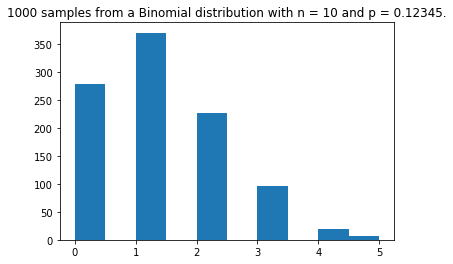In :
m = 1000
n = 10
p = 0.5
X = [ cython_inversion_binomial(n, p) for _ in range(m) ]
plt.figure()
plt.hist(X)
plt.title(f"{m} samples from a Binomial distribution with n = {n} and p = {p}.")
plt.show()

Out:
<Figure size 432x288 with 0 Axes>
Out:
(array([  2.,   6.,  37., 105., 205., 234., 244., 126.,  33.,   8.]),
array([ 0.,  1.,  2.,  3.,  4.,  5.,  6.,  7.,  8.,  9., 10.]),
<a list of 10 Patch objects>)
Out:
Text(0.5, 1.0, '1000 samples from a Binomial distribution with n = 10 and p = 0.5.')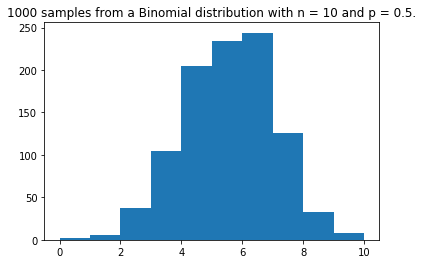In :
inversion_binomialm = 1000
n = 10
p = 0.98765
X = [ cython_inversion_binomial(n, p) for _ in range(m) ]
plt.figure()
plt.hist(X)
plt.title(f"{m} samples from a Binomial distribution with n = {n} and p = {p}.")
plt.show()

Out:
<Figure size 432x288 with 0 Axes>
Out:
(array([  7.,   0.,   0.,   0.,   0.,  99.,   0.,   0.,   0., 894.]),
array([ 8. ,  8.2,  8.4,  8.6,  8.8,  9. ,  9.2,  9.4,  9.6,  9.8, 10. ]),
<a list of 10 Patch objects>)
Out:
Text(0.5, 1.0, '1000 samples from a Binomial distribution with n = 10 and p = 0.98765.')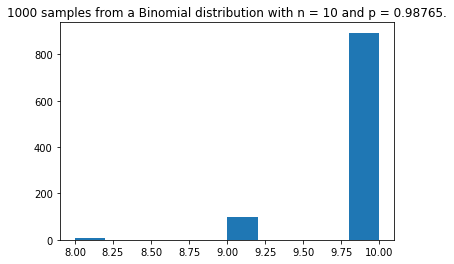## Numerical experiments to check time cost of the different versions¶

In :
n = 100

In :
naive_binomial
first_inversion_binomial
inversion_binomial
cython_inversion_binomial
numpy_binomial

Out:
<function __main__.naive_binomial(n:int, p:float) -> int>
Out:
<function __main__.first_inversion_binomial(n:int, p:float) -> int>
Out:
<function __main__.inversion_binomial(n:int, p:float) -> int>
Out:
<function _cython_magic_beb04c003b39b7afc4e8a1cfd3d3afad.cython_inversion_binomial>
Out:
<function __main__.numpy_binomial(n:int, p:float) -> int>

We can use the %timeit magic to check the (mean) computation time of all the previously mentioned functions:

In :
%timeit naive_binomial(n, 0.123456)
%timeit first_inversion_binomial(n, 0.123456)
%timeit inversion_binomial(n, 0.123456)
%timeit cython_inversion_binomial(n, 0.123456)
%timeit numpy_binomial(n, 0.123456)

21.8 µs ± 832 ns per loop (mean ± std. dev. of 7 runs, 10000 loops each)
320 µs ± 9.6 µs per loop (mean ± std. dev. of 7 runs, 1000 loops each)
2.45 µs ± 154 ns per loop (mean ± std. dev. of 7 runs, 100000 loops each)
201 ns ± 31 ns per loop (mean ± std. dev. of 7 runs, 10000000 loops each)
2.4 µs ± 307 ns per loop (mean ± std. dev. of 7 runs, 100000 loops each)


Apparently, our cython method is faster than the function from numpy!

We also check that our first naive implementation of the inversion method was suboptimal, as announced, because of its pre computation of all the values of $\mathbb{P}(X=k)$. However, we check that the naive method, using the sum of $n$ binomial samples, is as comparably efficient to the pure-Python inversion-based method (for this small $n=100$).

In :
%timeit naive_binomial(n, 0.5)
%timeit first_inversion_binomial(n, 0.5)
%timeit inversion_binomial(n, 0.5)
%timeit cython_inversion_binomial(n, 0.5)
%timeit numpy_binomial(n, 0.5)

21.6 µs ± 1.38 µs per loop (mean ± std. dev. of 7 runs, 10000 loops each)
311 µs ± 16.8 µs per loop (mean ± std. dev. of 7 runs, 1000 loops each)
7.86 µs ± 168 ns per loop (mean ± std. dev. of 7 runs, 100000 loops each)
309 ns ± 5.56 ns per loop (mean ± std. dev. of 7 runs, 1000000 loops each)
2.08 µs ± 319 ns per loop (mean ± std. dev. of 7 runs, 1000000 loops each)

In :
%timeit naive_binomial(n, 0.987654)
%timeit first_inversion_binomial(n, 0.987654)
%timeit inversion_binomial(n, 0.987654)
%timeit cython_inversion_binomial(n, 0.987654)
%timeit numpy_binomial(n, 0.987654)

21 µs ± 629 ns per loop (mean ± std. dev. of 7 runs, 10000 loops each)
298 µs ± 6.24 µs per loop (mean ± std. dev. of 7 runs, 1000 loops each)
777 ns ± 22.1 ns per loop (mean ± std. dev. of 7 runs, 1000000 loops each)
208 ns ± 7.2 ns per loop (mean ± std. dev. of 7 runs, 1000000 loops each)
1.52 µs ± 56 ns per loop (mean ± std. dev. of 7 runs, 1000000 loops each)


It's quite awesome to see that our inversion-based method is more efficient that the numpy function, both in the pure-Python and the Cython versions! But it's weird, as the numpy function is... based on the inversion method, and itself written in C!

See the source code, numpy/distributions.c line 426 (on the 28th February 2019, commit 7c41164).

But the trick is that the implementation in numpy uses the inversion method (running in $\Omega(np)$) if $pn < 30$, and a method denoted "BTPE" otherwise. I need to work on this method! The BTPE algorithm is much more complicated, and it is described in the following paper:

Kachitvichyanukul, V.; Schmeiser, B. W. (1988). "Binomial random variate generation". Communications of the ACM. 31 (2): 216–222. doi:10.1145/42372.42381.

See the source code, numpy/distributions.c line 263 (on the 28th February 2019, commit 7c41164).

## Checking that sampling from $Bin(n,p)$ requires a time $\Omega(n)$.¶

In :
n = 100
%timeit naive_binomial(n, random.random())
%timeit inversion_binomial(n, random.random())
%timeit cython_inversion_binomial(n, random.random())
%timeit numpy_binomial(n, random.random())

22.3 µs ± 1.26 µs per loop (mean ± std. dev. of 7 runs, 10000 loops each)
4.83 µs ± 570 ns per loop (mean ± std. dev. of 7 runs, 100000 loops each)
412 ns ± 70.9 ns per loop (mean ± std. dev. of 7 runs, 1000000 loops each)
1.95 µs ± 173 ns per loop (mean ± std. dev. of 7 runs, 1000000 loops each)

In :
n = 1000
%timeit naive_binomial(n, random.random())
%timeit inversion_binomial(n, random.random())
%timeit cython_inversion_binomial(n, random.random())
%timeit numpy_binomial(n, random.random())

228 µs ± 3.58 µs per loop (mean ± std. dev. of 7 runs, 1000 loops each)
44 µs ± 2.41 µs per loop (mean ± std. dev. of 7 runs, 10000 loops each)
859 ns ± 21.2 ns per loop (mean ± std. dev. of 7 runs, 1000000 loops each)
1.83 µs ± 201 ns per loop (mean ± std. dev. of 7 runs, 1000000 loops each)

In [ ]:
n = 10000
%timeit naive_binomial(n, random.random())
%timeit inversion_binomial(n, random.random())
%timeit cython_inversion_binomial(n, random.random())
%timeit numpy_binomial(n, random.random())

2.62 ms ± 238 µs per loop (mean ± std. dev. of 7 runs, 100 loops each)


As we can see, our inversion method (no matter the implementation) runs in $O(n)$ (for $p$ in average $1/2$ in the trials above). But numpy's implementation is using the BTPE method, which runs in $O(1)$.